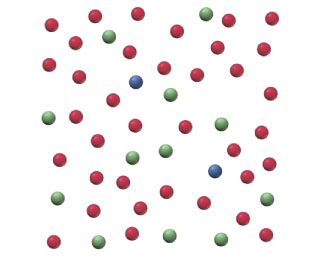# Problem: Below is a representation of 50 atoms of a fictitious element called westmontium (Wt). The red spheres represent Wt-296, the blue spheres Wt-297, and the green spheres Wt-298. Assuming that the sample is statistically representative of a naturally occurring sample, calculate the percent natural abundance of Wt-296 isotope.

###### FREE Expert Solution

In this problem, we asked to find the percent natural abundance of Wt-296 isotope.

Recall that isotopes are elements with the same number of protons, but with different number of neutrons.

We are given the following representation of the 50 atoms of the fictitious element called westmontium (Wt).

91% (98 ratings)###### Problem Details

Below is a representation of 50 atoms of a fictitious element called westmontium (Wt). The red spheres represent Wt-296, the blue spheres Wt-297, and the green spheres Wt-298.Assuming that the sample is statistically representative of a naturally occurring sample, calculate the percent natural abundance of Wt-296 isotope.